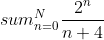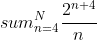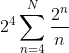# What is the summation of this partial sequence?

• Amaelle
Besides convergence of the Taylor series, you're trying to calculate a finite sum whereas the Taylor series is an infinite sum.$$\sum_{k=4}^{N+4} \frac{x^k}{k} = \sum_{k=4}^{N+4} \int x^{k-1}\,dx$$$$\sum_{k=4}^{N+4} \frac{x^k}{k} = \sum_{k=4}^{N+4} \int x^{k-1}\,dx$$The Taylor series for ##x## is:$$\displaystyle \sum_{n=0}^N \ {2^nf #### Amaelle Homework Statement look at the image Relevant Equations Sommation taylor series Greetings! I want to caluculate the summation of this following serieI started by removing the 4 byand thenand I thought of the taylor expansion of Log(1-x)=-∑xn/n but as the 2 is not inside (-1,1) I couldn´t use it any hint? thank you! Best ! I started by removing the 4 That is derailing spectacularly ! Write down a few terms of the one and the other and see that the two are very different ! Also: renaming an index (##n## here) works better if you use a different symbol (e.g. use ##k = n+4##) and that changes the summation limits to: ##n=0,\quad k = n +4 \Rightarrow ## lower limit is ##k = 4## ##n=N,\quad k = n+4 \Rightarrow ## upper limit is ##k = N+4## And in the numerator you have ##n=k-4## so the exponent is not ##k## but ##k-4## ##\ ## Homework Statement:: look at the image Relevant Equations:: Sommation taylor series Greetings! I want to caluculate the summation of this following serie View attachment 297263 I started by removing the 4 by View attachment 297264 and then View attachment 297265 and I thought of the taylor expansion of Log(1-x)=-∑xn/n but as the 2 is not inside (-1,1) I couldn´t use it any hint? thank you! Best ! you are right thank you and then? the main problem remain unsolved? If you have already studied calculus, you may make use of the relation $$\int_{-\infty}^1 2^{mt} dt = [\frac{2^{mt}}{m \ln 2}]_{-\infty}^1=\frac{1}{\ln 2}\frac{2^m}{m}$$ for easy summation. •Amaelle If you have already studied calculus, you may make use of the relation $$\int_{-\infty}^1 2^{mt} dt = [\frac{2^{mt}}{m \ln 2}]_{-\infty}^1=\frac{1}{\ln 2}\frac{2^m}{m}$$ for easy summation. thank you! the main problem remain unsolved Haha, if you're starting in the wrong direction it won't be solved anywayI don't have all the answers but a modest beginning might come about by writing down a bunch of terms for a low value of ##N## ... Homework Statement:: look at the image Relevant Equations:: Sommation taylor series I want to caluculate So the actual homework statement is Homework Statement:: 'Calculate (or: find a compact expression for) ##\quad \displaystyle \sum_{n=0}^N \ {2^n\over n+4}\quad ## '  Ah, we have another contributor ! ##\ ## •Amaelle Haha, if you're starting in the wrong direction it won't be solved anywayI don't have all the answers but a modest beginning might come about by writing down a bunch of terms for a low value of ##N## ... Indeed, that was a huge contribution! I can do this kind of deadly mistakes during exams, thanks a million for point it out! I doubt you will see this problem on an exam. The solution is rather difficult resulting in a complex valued function involving the Lerch transcendent. I'm surprised that it was issued as a homework problem (that's just mean). •Delta2 and Amaelle I doubt you will see this problem on an exam. The solution is rather difficult resulting in a complex valued function involving the Lerch transcendent. I'm surprised that it was issued as a homework problem (that's just mean). Indeed, my instructor said he can asked it in the exam, so I tried to solved it, :) I thought of the taylor expansion of Log(1-x)=-∑xn/n but as the 2 is not inside (-1,1) I couldn´t use it Besides convergence of the Taylor series, you're trying to calculate a finite sum whereas the Taylor series is an infinite sum. Here's a hint:$$\sum_{k=4}^{N+4} \frac{x^k}{k} = \sum_{k=4}^{N+4} \int x^{k-1}\,dx Remember that you're evaluating a finite sum, so the problem actually isn't too bad.

•Amaelle and BvU